### Home > CALC > Chapter 2 > Lesson 2.1.1 > Problem2-7

2-7.
1. Consider the following function: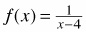Homework Help ✎

1. Sketch a graph of the function. Label any holes or asymptotes.

2. Find the inverse of f(x). Is the inverse a function? Why or why not?

3. Sketch a graph of the inverse and compare this sketch to the graph of f(x) from part (a).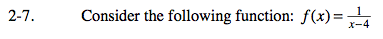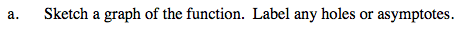No holes. Two asymptotes: x = 4 and y = 0. Label them.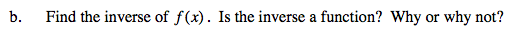$y=\frac{1}{x-4}\ \ \ \ \ \text{Rename }f(x)\text{ as }y$

$y(x-4)=1 \ \ \ \text{solve for }x$

$x-4=\frac{1}{y}$

$x=\frac{1}{y}+4$

$f^{-1}(x)=\frac{1}{x}+4 \ \ \ \text{Switch the }x \text{ and }y$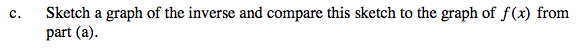You do not need to know the inverse equation to sketch a graph of the inverse. Start by switching the horizontal and vertical asymptotes. Then plot some key points, and connect.

Use the eTool below to explore the graphs.
Click on the link to the right to view the full version of the eTool. Calc 2-7 HW eTool